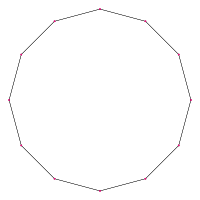# Dodecagon

﻿
Dodecagon
Regular dodecagonEdges and vertices 12
Schläfli symbols {12}
Coxeter–Dynkin diagramsSymmetry group Dihedral (D12)
Internal angle
(degrees)
150°

In geometry, a dodecagon is any polygon with twelve sides and twelve angles.

## Regular dodecagon

It usually refers to a regular dodecagon, having all sides of equal length and all angles equal to 150°. Its Schläfli symbol is {12}.

The area of a regular dodecagon with side a is given by:\begin{align} A & = 3 \cot\left( \frac{\pi}{12} \right) a^2 = 3 \left( 2+\sqrt{3} \right) a^2 \\ & \simeq 11.19615242\,a^2. \end{align}

Or, if R is the radius of the circumscribed circle,$A = 6 \sin\left( \frac{\pi}{6}\right) R^2 = 3 R^2.$

And, if r is the radius of the inscribed circle,\begin{align} A & = 12 \tan\left( \frac{\pi}{12}\right) r^2 = 12 \left( 2-\sqrt{3} \right) r^2 \\ & \simeq 3.2153903\,r^2. \end{align}

A simple formula for area (given the two measurements) is:$\ A = 3ad$ where d is the distance between parallel sides.

Length d is the height of the dodecahedron when it sits on a side as base, and the diameter of the inscribed circle.

By simple trigonometry, d = a(1 + 2cos30° + 2cos60°).

## Dodecagon construction

A regular dodecagon is constructible using compass and straightedge:Construction of a regular dodecagon

## Occurrence

Here are 3 example periodic plane tilings that use dodecagons:

### Petrie polygons

The regular dodecagon is the Petrie polygon for many higher dimensional polytopes, seen as orthogonal projections in Coxeter planes, including:

## Examples in use

In block capitals, the letters E and H (and I in a slab serif font) have dodecagonal outlines.

Regular dodecagonal coins include:

Wikimedia Foundation. 2010.

### Look at other dictionaries:

• dodecagon — DODECAGÓN, dodecagoane, s.n. Poligon cu douăsprezece laturi (latură) şi douăsprezece unghiuri. – Din fr. dodécagone. Trimis de claudia, 13.09.2007. Sursa: DEX 98 ﻿ dodecagón s. n., pl. dodecagoáne Trimis de siveco, 10.08.2004. Sursa: Dicţionar… …   Dicționar Român

• Dodecagon — Do*dec a*gon, n. [Gr. ? twelve + ? angle: cf. F. dod[ e]cagone.] (Geom.) A figure or polygon bounded by twelve sides and containing twelve angles. [1913 Webster] || …   The Collaborative International Dictionary of English

• dodecagon — ► NOUN ▪ a plane figure with twelve straight sides and angles …   English terms dictionary

• dodecagon — [dō dek′ə gän΄, dō dek′əgən] n. [Gr dōdekagōnon: see DODECA & GON] a plane figure with twelve angles and twelve sides …   English World dictionary

• dodecagon —    A closed shape bounded by twelve straight line segments. The formula with which to find an equilateral dodecagon s area is 11.1961 times the length of one side squared. Also see circle, dodecahedron, mathematics, polygon, radial, shape, and… …   Glossary of Art Terms

• dodecagon — noun Etymology: Greek dōdekagōnon, from dōdeka + gōnon gon Date: circa 1658 a polygon of 12 angles and 12 sides …   New Collegiate Dictionary

• dodecagon — dodecagonal /doh de kag euh nl/, adj. /doh dek euh gon , geuhn/, n. Geom. a polygon having 12 angles and 12 sides. Also, duodecagon. [1650 60; DODECA + GON] * * * …   Universalium

• dodecagon — noun a polygon with twelve edges and twelve angles Syn: duodecagon See Also: dodecagonal …   Wiktionary

• dodecàgon — do|de|cà|gon Mot Pla Nom masculí dodecasíl·lab do|de|ca|síl|·lab Mot Pla Adjectiu variable dodecasíl·lab do|de|ca|síl|·lab Mot Pla Nom masculí …   Diccionari Català-Català

• dodecagón — s. n., pl. dodecagoáne …   Romanian orthography